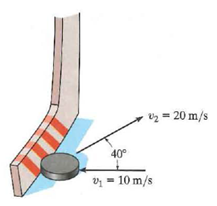# Problem: A hockey puck is traveling to the left with a velocity of v1 =10 m/s when it is struck by a hockey stick and given a velocity of v2 =20 m/s as shown. Determine the magnitude of the net impulse exerted by the hockey stick on the puck. The puck has a mass of 0.2 kg

###### FREE Expert Solution

Impulse is expressed as:

$\overline{)\begin{array}{rcl}\mathbf{I}\mathbf{m}\mathbf{p}\mathbf{u}\mathbf{l}\mathbf{s}\mathbf{e}& {\mathbf{=}}& {\mathbf{F}}_{\mathbf{a}\mathbf{v}}\mathbf{∆}\mathbf{t}\\ & {\mathbf{=}}& \mathbf{m}\mathbf{∆}\mathbf{v}\end{array}}$, m is the mass of the hockey puck and Δv is the change in velocity.

The initial velocity is:

v0 = v1i

Let's take velocity to the right to be positive and to the left to be negative.

91% (492 ratings)###### Problem Details

A hockey puck is traveling to the left with a velocity of v1 =10 m/s when it is struck by a hockey stick and given a velocity of v2 =20 m/s as shown. Determine the magnitude of the net impulse exerted by the hockey stick on the puck. The puck has a mass of 0.2 kgFrequently Asked Questions

What scientific concept do you need to know in order to solve this problem?

Our tutors have indicated that to solve this problem you will need to apply the Momentum & Impulse in 2D concept. You can view video lessons to learn Momentum & Impulse in 2D. Or if you need more Momentum & Impulse in 2D practice, you can also practice Momentum & Impulse in 2D practice problems.